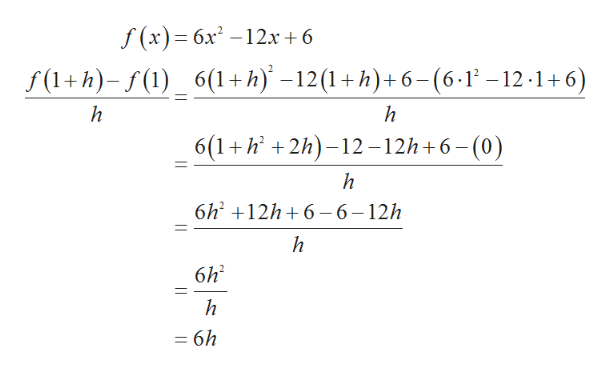# Let f(x) be the function 6x^2−12x+6. Then the quotient(f(1+h)-f(1))/h can be simplified to ah+b for:a=b=

Question
16 views

Let f(x) be the function 6x^2−12x+6. Then the quotient
(f(1+h)-f(1))/h can be simplified to ah+b for:
a=

b=

check_circle

Step 1

Consider the given function,

Step 2

Substitute the value of f(x) as per the given expression (f(1...help_outlineImage Transcriptionclosef(x)= 6x2 -12x +6 f(1+h)-f(1) 6(1+h) -12(1+h)+6-(6-1-12-1+6) h 6(1 h2h-12 -12h+6-(0) h 6h2 12h6 - 6-12h h 6h2 = 6h fullscreen

### Want to see the full answer?

See Solution

#### Want to see this answer and more?

Solutions are written by subject experts who are available 24/7. Questions are typically answered within 1 hour.*

See Solution
*Response times may vary by subject and question.
Tagged in

### Calculus